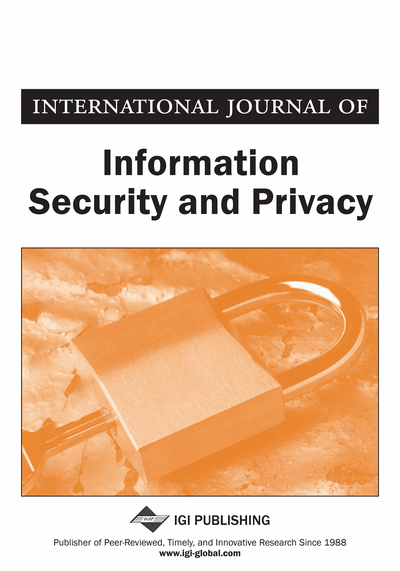# Computational Complexity Analysis for a Class of Symmetric Cryptosystems Using Simple Arithmetic Operations and Memory Access Time

Walid Y. Zibideh (Qualcomm, San Diego, CA, USA) and Mustafa M. Matalgah (Department of Electrical Engineering, University of Mississippi, University, MS, USA)
DOI: 10.4018/jisp.2013010105
Available
\$29.50
No Current Special Offers

## Abstract

A secure cryptosystem could be very complicated, time consuming and hard to implement. Therefore, the complexity of the cryptosystem should be taken into account during design and implementation. In this work, the authors introduce a comprehensive and platform independent complexity analysis for a class of symmetric block cryptosystems, by which it will be easier to evaluate the performance of some used cryptosystems. Previous works lacked the comprehensiveness in their analysis, due to the fact that the memory access time was completely ignored, which greatly degrades the accuracy of the analysis and limits it to one data block only. In this paper the authors analytically compute the complexity for a class of symmetric cryptosystems in terms of the number of the clock cycles required and in terms of the required time for encryption/decryption, independently of the hardware or software used in the encryption/decryption process. Moreover, this is the first complexity analysis that considers the required time to access and retrieve information from memory, which makes the analysis more comprehensive and accurate than previous work as well as being general for any number of encryption data blocks. In addition, computer simulations are used to truly evaluate the accuracy of the analysis and to show how the analytical results match the simulation results.
Article Preview
Top

## Analytical Complexity Analysis

In this section we provide analytical derivation for the time required for encryption using different symmetric block cryptosystems. We perform the analysis for DES, 3-DES, AES and M-DES cryptosystems. In this analysis, we will break down the operations performed on the data for each cryptosystem into simple byte-wise AND, OR, XOR, Shift and memory access operations. In general, the total required time to encrypt one block of data is given by the number of operations that are required to encrypt this data block multiplied by the clock cycle time for each operation. The following equation gives a general method for computing the total time required to encrypt one data block using any cryptosystem

(1) whereis the total encryption time for one data block,andare the number of clock cycles required to perform one byte-wise XOR, AND, OR, Shift and memory access operations respectively. Andare the clock cycle times for one byte-wise XOR, AND, OR, Shift and memory access operations respectively.

## Complete Article List

Search this Journal:
Reset
Volume 17: 1 Issue (2023)
Volume 16: 4 Issues (2022): 2 Released, 2 Forthcoming
Volume 15: 4 Issues (2021)
Volume 14: 4 Issues (2020)
Volume 13: 4 Issues (2019)
Volume 12: 4 Issues (2018)
Volume 11: 4 Issues (2017)
Volume 10: 4 Issues (2016)
Volume 9: 4 Issues (2015)
Volume 8: 4 Issues (2014)
Volume 7: 4 Issues (2013)
Volume 6: 4 Issues (2012)
Volume 5: 4 Issues (2011)
Volume 4: 4 Issues (2010)
Volume 3: 4 Issues (2009)
Volume 2: 4 Issues (2008)
Volume 1: 4 Issues (2007)
View Complete Journal Contents Listing